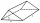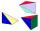# Solid geometry, stereometry

Solid geometry is the name for the geometry of three-dimensional Euclidean space.

Stereometry deals with the measurements of volumes of various solid figures (three-dimensional figures) including pyramids, prisms and other polyhedrons; cylinders; cones; truncated cones; and balls bounded by spheres.

#### Number of problems found: 961

• Cube wallsFind the volume and surface area of the cube if the area of one wall is 40cm2.
• The roomThe room has a cuboid shape with dimensions: length 50m and width 60dm and height 300cm. Calculate how much this room will cost paint (floor is not painted) if the window and door area is 15% of the total area and 1m2 cost 15 euro.
• Paper boxCalculate how much we'll pay for a three-side shaped prism box with a triangular base, and if it measures 12cm and 1.6dm, the hypotenuse measures 200mm. The box is 34cm high. We pay 0,13 € per square meter of paper.
• Axial cutThe cone surface is 388.84 cm2, the axial cut is an equilateral triangle. Find the cone volume.
• Pyramid 8Calculate the volume and the surface area of a regular quadrangular pyramid with the base side 9 cm and side wall with the base has an angle 75°.Calculate the surface area and volume of a regular quadrangular pyramid: sides of bases (bottom, top): a1 = 18 cm, a2 = 6cm angle α = 60 ° (Angle α is the angle between the side wall and the plane of the base.) S =? , V =?
• CostumeDenisa is preparing for a goldsmith's costume carnival. During the preparations, she thought she would let her hair wipe instead - she would apply a 5 μm thick layer of gold to each hair. How much gold would Denisa need? Assume that all hundred thousand DCalculate the volume and surface area of a regular quadrangular prism 35 cm high and the base diagonal of 22 cm.
• PyramidCuboid ABCDEFGH has dimensions AB 3 cm, BC 4 cm, CG 5 cm. Calculate the volume and surface area of a triangular pyramid ADEC.
• The potDiameter of the pot 38 cm. The height is 30 cm. How many liters of water can fit in the pot?
• RainfallHow many liters of water did fell in a 32m long and 8m wide garden, if 8mm of rain fell?
• Water channelThe cross section of the water channel is a trapezoid. The width of the bottom is 19.7 m, the water surface width is 28.5 m, the side walls have a slope of 67°30' and 61°15'. Calculate how much water flows through the channel in 5 minutes if the water flo
• Pyramid 4sidesCalculate the volume and the surface of a regular quadrangular pyramid when the edge of the base is 4 cm long and the height of the pyramid is 7 cm.
• Square pyramidCalculate the volume of the pyramid with the side 5cm long and with a square base, side-base has angle of 60 degrees.
• Cube cutIn the ABCDA'B'C'D'cube, it is guided by the edge of the CC' a plane witch dividing the cube into two perpendicular four-sided and triangular prisms, whose volumes are 3:2. Determine in which ratio the edge AB is divided by this plane.
• Cuboid and eq2Calculate the volume of cuboid with square base and height 6 cm if the surface area is 48 cm2.4 m long ladder touches the cube 1mx1m at the wall. How high reach on the wall?
• Prism - eq triangleCalculate the volume and surface of the prism with the base of an equilateral triangle with side a = 4cm and the body height is 6cm.
• Hexagon rotationA regular hexagon of side 6 cm is rotated through 60° along a line passing through its longest diagonal. What is the volume of the figure thus generated?
• Pool in litresPool has a width of 3.5 m length of 6 m and a height 1.60 meters. Calculate pool volume in liters.

Do you have an interesting mathematical word problem that you can't solve it? Submit a math problem, and we can try to solve it.

We will send a solution to your e-mail address. Solved examples are also published here. Please enter the e-mail correctly and check whether you don't have a full mailbox.

Please do not submit problems from current active competitions such as Mathematical Olympiad, correspondence seminars etc...